# Transportation Problem

Use the solver in Excel to find the number of units to ship from each factory to each customer that minimizes the total cost.

### Formulate the Model

The model we are going to solve looks as follows in Excel.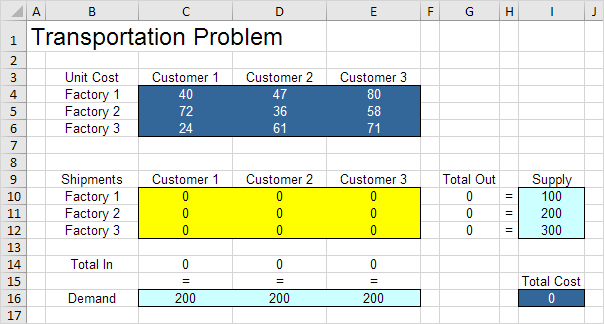1. To formulate this transportation problem, answer the following three questions.

a. What are the decisions to be made? For this problem, we need Excel to find out how many units to ship from each factory to each customer.

b. What are the constraints on these decisions? Each factory has a fixed supply and each customer has a fixed demand.

c. What is the overall measure of performance for these decisions? The overall measure of performance is the total cost of the shipments, so the objective is to minimize this quantity.

2. To make the model easier to understand, create the following named ranges.

Range Name Cells
UnitCost C4:E6
Shipments C10:E12
TotalIn C14:E14
Demand C16:E16
TotalOut G10:G12
Supply I10:I12
TotalCost I16

3. Insert the following functions.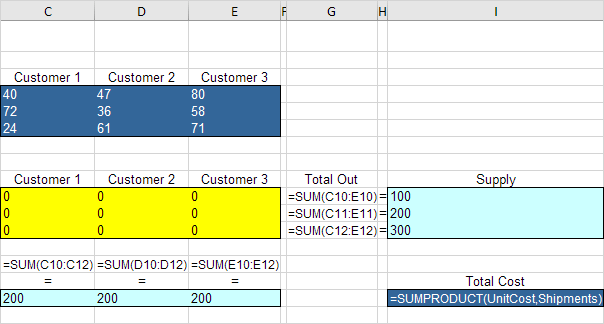Explanation: The SUM functions calculate the total shipped from each factory (Total Out) to each customer (Total In). Total Cost equals the sumproduct of UnitCost and Shipments.

### Trial and Error

With this formulation, it becomes easy to analyze any trial solution.

For example, if we ship 100 units from Factory 1 to Customer 1, 200 units from Factory 2 to Customer 2, 100 units from Factory 3 to Customer 1 and 200 units from Factory 3 to Customer 3, Total Out equals Supply and Total In equals Demand. This solution has a total cost of 27800.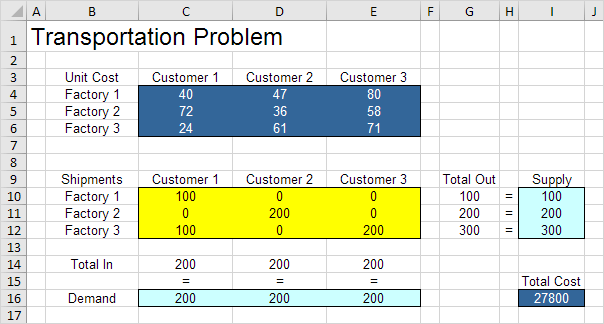It is not necessary to use trial and error. We shall describe next how the Excel Solver can be used to quickly find the optimal solution.

### Solve the Model

To find the optimal solution, execute the following steps.

1. On the Data tab, in the Analyze group, click Solver.Enter the solver parameters (read on). The result should be consistent with the picture below.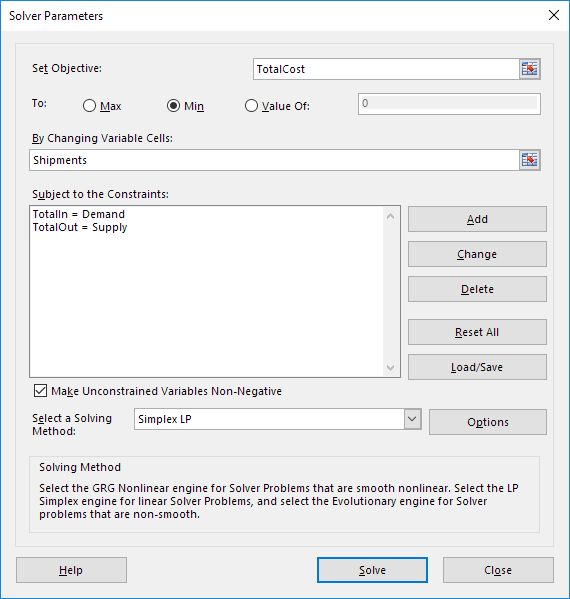You have the choice of typing the range names or clicking on the cells in the spreadsheet.

2. Enter TotalCost for the Objective.

3. Click Min.

4. Enter Shipments for the Changing Variable Cells.

5. Click Add to enter the following constraint.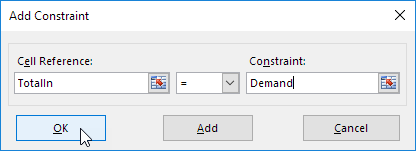6. Click Add to enter the following constraint.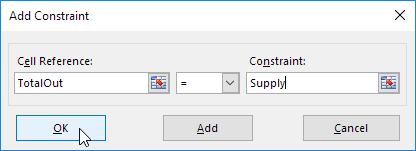7. Check 'Make Unconstrained Variables Non-Negative' and select 'Simplex LP'.

8. Finally, click Solve.

Result: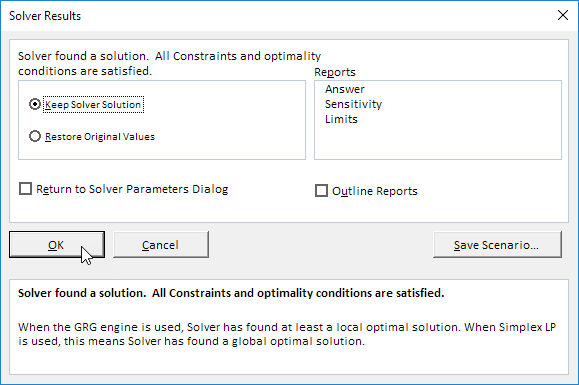The optimal solution: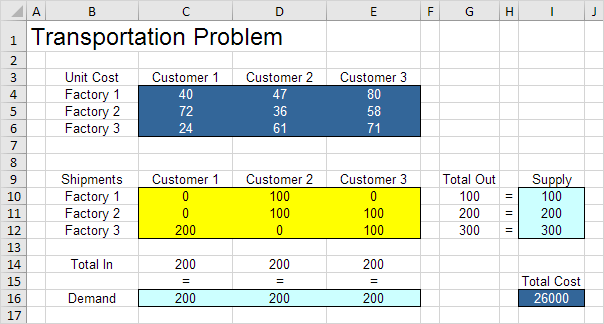Conclusion: it is optimal to ship 100 units from Factory 1 to Customer 2, 100 units from Factory 2 to Customer 2, 100 units from Factory 2 to Customer 3, 200 units from Factory 3 to Customer 1 and 100 units from Factory 3 to Customer 3. This solution gives the minimum cost of 26000. All constraints are satisfied.

Go to Next Chapter: Analysis ToolPak